# The Matrix: Red or Blue?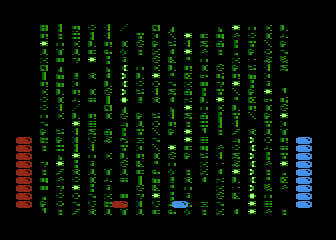This is a little game for the 2021 NOMAM's BASIC 10-liners Contest. This program fits in the "stock" PUR-120 category, and it was written in Turbo Basic XL for the 8-bits ATARI XL/XE. Development started on 2021-03-17, and it took 2 days. The final version's date is 2021-03-25.

UPDATE: It obtained the 4th place of 26 entries in the category.

## Description

Two people opted for different pills... which one will be able to enter The Matrix?

NOTE: This is a game for simultaneous two players, and two joysticks are required.

## Instructions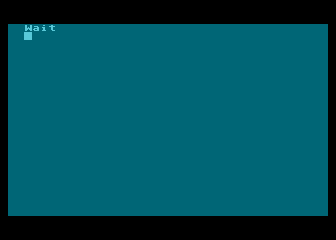Wait about 5 seconds while The Matrix is being loaded...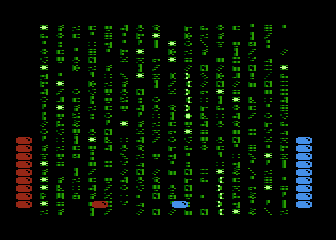Players are placed side by side. Use the joystick to move your pill though the Matrix code. Every pill can only be moved to the left or to the right.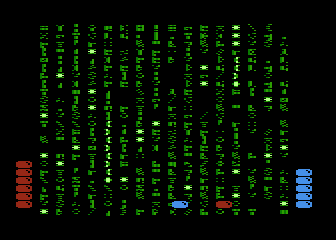Each player starts with ten pills. Avoid highlighted code! Being hit by those it will cost you a pill. Be careful, Mr. Smith's code is also highlighted and it appears anywere in a rush.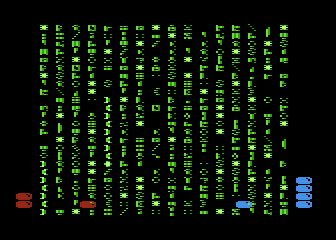At first, there won't be many highlighted chars, but they will increase as the time goes. The bright of the normal chars will also gradually increase, making it harder to identify the chars to avoid.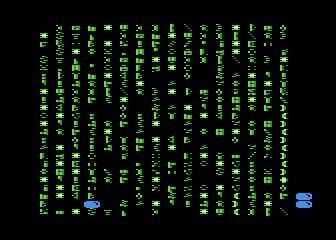If a player runs out of pills, The Matrix will be halted. The winner is the player with at least one pill. If there are no pills in the screen, it was a draw. Press RETURN key to compete again.

## Development of the game

When The Matrix: Red Pill was almost finished, I thought that a two players version could be written using the same code. Of course, it won't fit the PUR-80 category, but it could be moved to PUR-120 category.

To do that, I changed the variables related to the pill into arrays, and added a simple FOR-NEXT loop to check for each player at a time. I also reassigned the P/M objects to use P0 and P1 for the pills and P2 and P3 to the stacks, with P1 and P3 set in blue instead of red.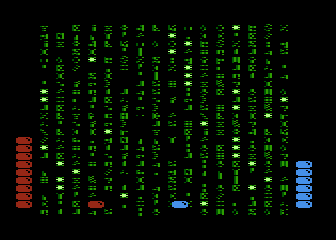Prototype of a two players version

The first prototype run OK and I was happy, but I used Turbo Basic XL interpreter to speed it up and mimic the single player version in Atari BASIC, as there is no restriction in the BASIC flavor in the PUR-120 category. The string variables were preserved, except that those 8K had to be moved from a base of 16K to 32K, because the interpreter of Turbo Basic XL runs in low memory instead of the high one of Atari BASIC ROM.

I was plenty of coding space, so I decided to add the game elements I had to drop in the single player version. That included hit sounds for each pill (two dedicated POKEY voices) and background sound (two voices with similar pitch to produce a waveform).

As an experiment, I decided to remove all the string variables and use fixed memory instead, with the use of POKE and MOVE instead of string operations to move screen and P/M data. The resulting code was smaller and a bit faster.

One of the extra effects I wanted for the original version, was some extra chars falling in front of the others, and I included it with an extra sound effect, which shares a POKEY voice with the background sound. Finally, I included other feature that I couldn't fit in the original version: a single POKE to increase the bright of one playfield to gradually make more difficult to identify the highlighted chars.

DISCLAIMER: This game is based on characters created by the Wachowski sisters, and it was developed without their supervision.

Get the REDBLUE.ATR file and set it as drive 1 in a real Atari (or emulator). Turn on the computer and the game should start after loading. Two joysticks are required.

A note for NTSC users: This game was programmed for PAL computers. If you want to play in NTSC systems, you can LOAD and RUN a modified copy of the game called "NTSC.BAS" that it is also included in the ATR file.

## The code

The abbreviated BASIC code is the following: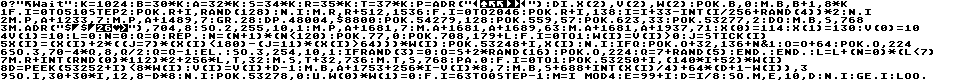The full and expanded BASIC listing is:

 The Matrix - Red or Blue? (c) 2021 by Victor Parada G. ```print "Wait" ``` Prints a message to manage anxiety ```k=1024 ``` Defines a constant to save source code space ```b=30*k a=32*k s=34*k r=35*k t=37*k ``` Reserves 8K of memory for game elements data, starting from 30720 2K: Blank data (used to initialize other string variables) 2K: Manages P/M data (2K for single scanline resolution) 1K: Screen data (forcing Display List to point here) 2K: Precalculated random data (8 blocks of 256 bytes with different density of obstacles for 8 levels) 1K: Temporary buffer to create next frame ```dim x(2),v(2),w(2) ``` Properties of each pill X(): Horizontal position of the pill V(): Number of available pills W(): Flags if there are pills available ```poke b,0 move b,b+1,8*k ``` Initializes all data blocks ```p=adr("{binary data}") ``` Bitmap of a pill ```for i=0 to 510 step 2 poke r+i,rand(128) next i ``` Initializes only 25% of random data (for setup speed) ```move r,r+512,1536 ``` Initializes the remaining 75% of random data by copying the first 25% ```for i=0 to 2046 ``` Puts the obstacles over the random data ``` poke r+i,138 ``` Puts an obstacle with high bit set (inverse video to enable PF4) ``` i=i+33-int(i/256+rand(4))*2 next i ``` Skips some bytes of data by jumping over based on the current zone ```move p,a+1233,7 move p,a+1489,7 ``` Puts a pill in the bottom of P0 and P1 ```graphics 28 ``` Sets screen to graphics mode 12 (ANTIC 4) without text window ```dpoke 48004,\$8800 ``` Changes Display List pointer to screen buffer ```poke 54279,128 ``` Sets PMBASE to P/M buffer ```poke 559,57 ``` Sets SDMCTL=1+32+8+16: One line resolution players only (no missiles) in narrow playfield ```poke 623,33 ``` Sets GPRIOR=1+32: Players over playfield and overlap of players have 3rd color ```poke 53277,2 ``` Sets GRACTL=2: Players only, no missiles P0: Red Pill P1: Blue Pill P2: Red stack P3: Blue stack ```do ``` MAIN LOOP ``` move b,s,768 ``` Clears the screen memory ``` move adr("{binary data}"),704,8 ``` Colors Red Pill: PAL=36 NTSC=52 Blue Pill: PAL=136 NTSC=152 Green fonts: PAL=178,182,0,190 ``` sound 2,255,10,1 ``` Enables a background sound ``` move p,a+1681,7 move a+1681,a+1689,63 move a+1681,a+1937,71 ``` Builds both stacks of pills on P2 and P3 ``` x(0)=114 x(1)=130 ``` Sets initial horizontal position of the pills near the middle of the playfield ``` v(0)=10 v(1)=10 ``` Total number of pills (lives) ``` l=0 ``` Initial density level ``` n=0 ``` Counter for steps in the level ``` q=0 ``` Falling char counter ``` repeat ``` GAME LOOP ``` n=(n+1)*(n<120) ``` Increases the number of level steps (reset to zero for next step) ``` poke 77,0 ``` Resets the attract mode counter ``` poke 708,179+l ``` Increases the bright of PF0 to make more difficult to see the highlighted chars on higher levels ``` for i = 0 to 1 ``` Checks both players for horizontal movement ``` w(i)=v(i)>0 ``` Checks for remaining pills ``` j=stick(i) ``` Reads the joystick ``` x(i)=(x(i)+2*((j=7)*(x(i)<180)-(j=11)*(x(i)>64)))*w(i) ``` Computes next horizontal position within limits, but removes it from screen if there are no more pills ``` poke 53248+i,x(i) ``` Moves P4 (the pill) to the next position ``` next i ``` ``` if q ``` Is there a falling char? ``` poke o+32,136+n&1 ``` Replaces the previous one with a trail ``` o=o+64 ``` Sets current position of the falling char ``` poke o,224 ``` Puts the falling char ``` sound 3,70-4*q,8,q/2 ``` Plays a sound ``` q=q-1 else ``` Decreases the counter for the falling char ``` sound 3,254,10,1 ``` Restores the background sound ``` if rand(3)=0 ``` Activates another falling char ``` o=s+2*rand(16) ``` Selects a column ``` poke o,224 ``` Puts a falling char ``` q=7+rand(5) endif endif ``` Sets the duration of the fall ``` l=l+(n=0)*(l<7) ``` Moves to next level if a reset in the number of steps is detected ``` move r+int(rnd(0)*112)*2+256*l,t,32 move s,t+32,736 ``` Prepares the next frame by joining of a line of random data and the current screen ``` move t,s,768 ``` Updates the screen from the temp buffer ``` pause 0 ``` Waits for a screen refresh ``` for i=0 to 1 ``` Checks each player for collisions ``` poke 53250+i,(140*i+52)*w(i) ``` Puts the column of remaining pills on screen, if there are any: ``` d=peek(53252+i)<8*w(i) ``` Repeats game loop if there is no collision between P4 and PF4 (and a pill is on screen) ``` v(i)=v(i)+d-1 ``` Decreases the number of pills ``` move b,a+1753+256*i-v(i)*8,7 ``` Removes a pill from the stack ``` move b,s+688+int(x(i)/4)+64*(d+1-w(i)),3 ``` Cleans the obstacle under the pill to avoid double collisions ``` sound i,30+30*i,12,8-d*8 ``` Buzzer when the pill hits a highlighted char ``` next i ``` ``` poke 53278,0 ``` Cleans the collision registers ``` until w(0)*w(1)=0 ``` End of GAME LOOP: Repeats if there are pills from both players ``` for i=63 to 0 step -1 m=i mod 4 e=99+i d=i/8 sound m,e,10,d next i ``` Bells and whistles! ``` get i ``` Waits for [RETURN] key to restart ```loop ``` End of MAIN LOOP